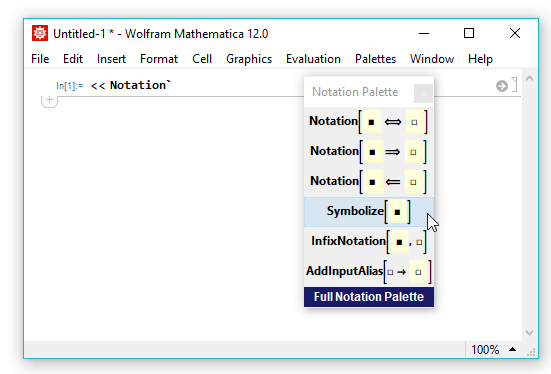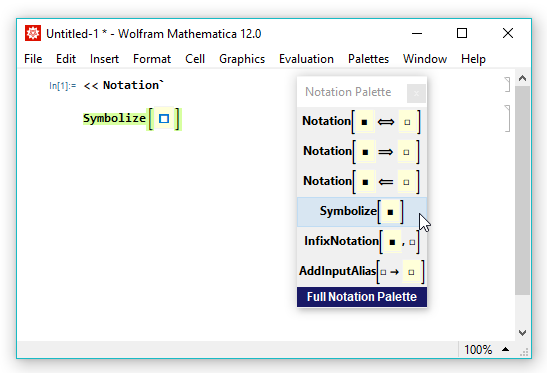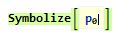# How do I define variables with subscripts or superscripts using the Notation Package?

In the Wolfram Language, attempting to define variables with subscripts can lead to errors. You can resolve any issues with defining such variables by using the function Symbolize in the Notation Package.

The Wolfram Language uses various syntactic rules to interpret input. In particular, expressions with subscripts are treated as functions of their components and not independent symbols. This can lead to recursion errors or other undesired behavior.

Example 1:

``````In := p = 2 p0

\$RecursionLimit::reclim2: Recursion depth of 1024 exceeded during evaluation of
Subscript[p, 0].

Out = Hold[2 p0]
``````

Example 2:

``````In := {x, xn} /. x->8
Out = {8, 8n}
``````

Expressions containing subscripts, superscripts or more general symbols can be specified as variables using the Symbolize function in the Notation Package.

1. Load the Notation Package into your Wolfram Language session using the command:
``<<Notation```

A window opens containing a truncated version of the Notation palette.2. Click the Symbolize button. The Symbolize function template is inserted into the current notebook, and the placeholder square is selected.3. Type or paste the expression you would like to use as a variable into the highlighted placeholder square.4. Evaluate the Symbolize expression with Shift+Enter.

The Symbolized expression is now interpreted as an atomic expression and not a function of its components.

``````In := p = 2 p0

Out = 2 p0

In := p2

Out = 4 p02
``````

Please note that when using the Notation Package and the Symbolize function, it is necessary to insert the function template from the relevant palette.

설명이 도움이 되었나요?

하실 말씀이 있습니까?

피드백 감사합니다.

## 지원 문의

청구서, 제품 동기화에 관한 질문에서 기술적인 질문까지 부담없이 문의하세요.전화하기

월요일 - 금요일
8am–5pm 중부 표준시

• 제품 등록 및 동기화
• 구매 전 정보 및 주문
• 설치 및 동작

## 고급 기술지원 (해당 고객을 대상으로)

월요일 - 목요일
8am–7pm 중부 표준시

금요일
8:30–10am & 11am–5pm 중부 표준시

• 우선적 기술지원
• Wolfram 전문가들의 제품 지원
• Wolfram 프로그래밍
• 고급 설치 지원